error: Content is protected !!

# MATHEMATICS: FORM ONE: Topic 3 - DECIMAL AND PERCENTAGE

TOPIC 3: DECIMAL AND PERCENTAGE

The Concept of Decimals
Explain the concept of decimals
A decimal- is defined as a number which consist of two parts separated by a point.The parts are whole number part and fractional part
Example 1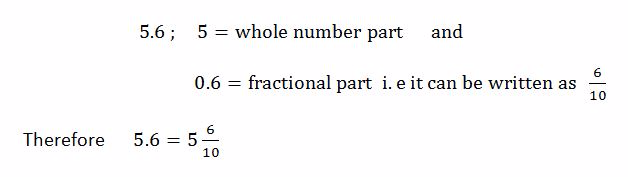Example 2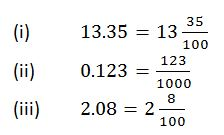Conversion of Fractions to Terminating Decimals and Vice Versa
Convert fractions to terminating decimals and vice versa
The first place after the decimal point is called tenths.The second place after the decimal point is called hundredths e.t.c
Consider the decimal number 8.152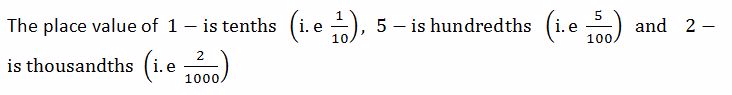NOTE
• To convert a fraction into decimal, divide the numerator by denominator
• To convert a decimal into fraction, write the digits after the decimal point as tenths, or hundredths or thousandths depending on the number of decimal places.
Example 3
Convert the following fractions into decimals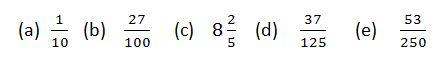Solution
Divide the numerator by denominator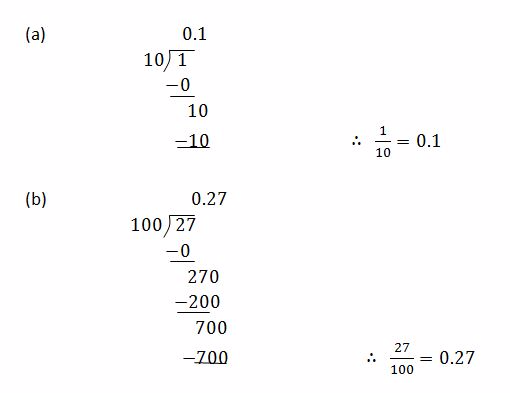Example 4
Evaluate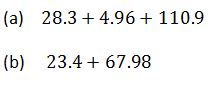Solution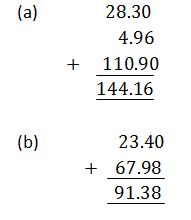Subtraction of Decimals
Subtract decimals
Example 5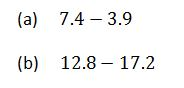Solution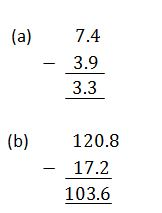Multiplication of Decimals
Multiply decimals
Example 6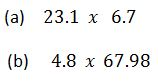Solution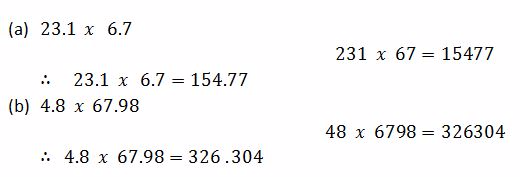Mixed Operations with Decimals
Perform mixed operations with decimals
Example 7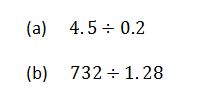Solution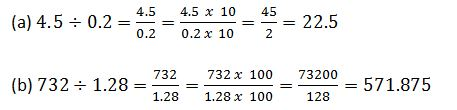Word Problems Involving Decimals
Solve word problems involving decimals
Example 8
If 58 out of 100 students in a school are boys, then write a decimal for the part of the school that consists of boys.
Solution
We can write a fraction and a decimal for the part of the school that consists of boys.
 fraction decimal 58/100 0.58

Expressing a Quantity as a Percentage
Express a quantity as a percentage
The percentage of a quantity is found by converting the percentage to a fraction or decimal and then multiply it by the quantity.
Example 9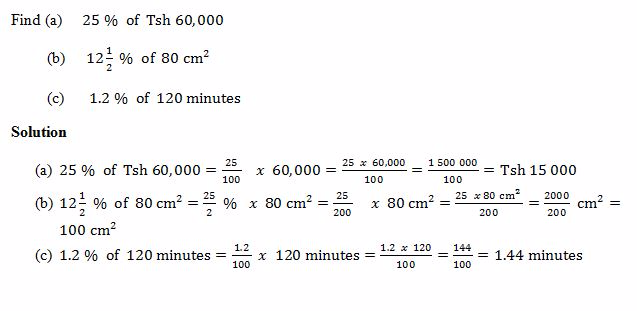NOTE:The concept of percentage of a quantity can be used to solve the problems involving percentage increase and decrease as shown in the below examples:-

A Fractions into Percentage and Vice Versa
Convert a fraction into percentage and vice versa
To change a fraction or a decimal into a percentage, multiply it by 100%
Example 10
Convert the following fractions into percentages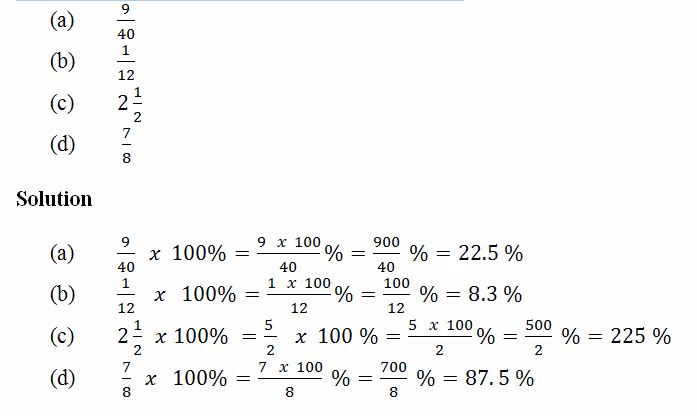A Decimal into Percentage and Vice Versa
Convert a decimal into percentage and vice versa
To change a percentage into a fraction or a decimal, divide it by 100%
Example 11
Convert the following percentages into decimals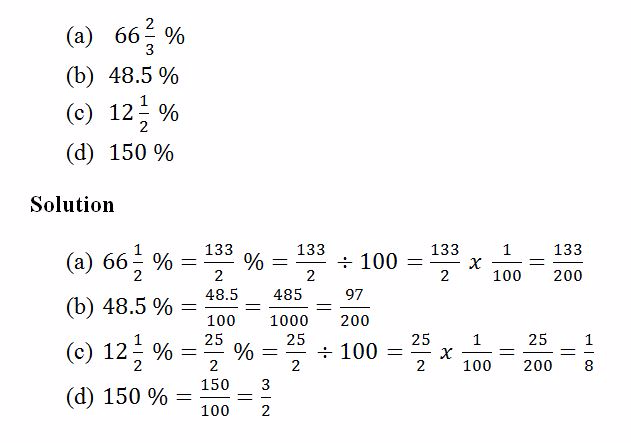Percentages in Daily Life
Apply percentages in daily life
Example 12
In an assignment, Regina scored 9 marks out of 12. Express this as a percentage
Solution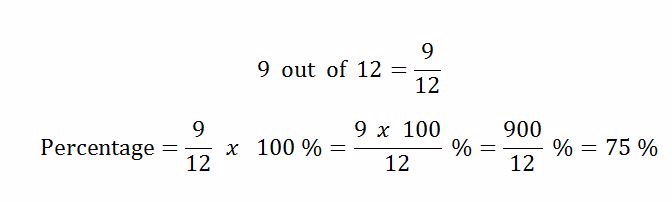Example 13
A school has 400 students of which 250 are girls. What percentage of the students are not girls?
Solution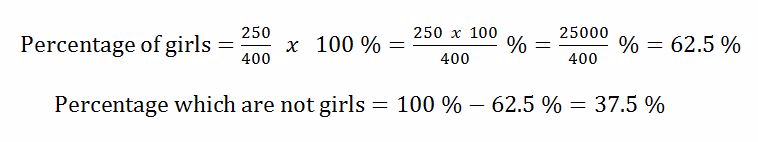1.2.3.